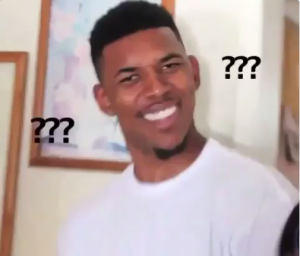# 2- stage DDM

Hi -

This questions has been discussed in another thread but I am not sure I understand as explained in the Schweser solutions (Exam 1 - Afternoon session solution).

Question:

Assume the Wansch Corporate is expected to pay a dividend of \$2.25 per share this year. Sales and profit for Wansch are forecasted to grow at a rate of 20% for two years after that, and then grow at 5% per year forever. Dividend and sales growth are expected to be equal. If Wansch’s shareholders require a 15% return, the per-share return value of Wansch’s common stock based on the DDM is closest to.

My solution is:

CF0 = \$2.25 is received at the end of the this current year (so no growth rate will be be applied to this divided but WILL be discounted back by 1 year to bring it back to PV)

At the end of current year (discussed above), we are still expected 2 years of 20% growth to the end-of-the-year dividend of \$2.25. This will take us to the end of year 3.

Therefore:

CF1 = \$2.25 * (1 + 0.20) = \$2.7 - this cash flow is due at the end of year 2 and will be discount back by 2 years

CF2 = \$2.25 * (1+ 0.20) * (1+0.20) = \$3.24 and will be discounted back by 3 years

Terminal Value:

Now we are expecting perpetual growth of 5%.

Therefore: the Present Value of the Terminal Value i.e. the value at the end of year 3 is:

Terminal Value = CF2 * (1+0.05)/(0.15-0.05) = \$3.24 * (1.05) / (0.10) = \$110.16

This terminal value will be discounted back by 3 years. Therefore, value of the stock is:

V = [ \$2.25 / (1+ 0.15) ] + [ \$2.7 / (1+ 0.15)2] + [\$3.24/(1.15)3] + [\$110.16/ (0.15 - 0.05) * (1.15)3]

= \$1.95 + \$2.04 + \$4.92 + \$951.6

= \$960.5

What is wrong with this approach? Am I interpreting the question incorrectly?

Schweser's upgraded learning platform and refreshed content are exactly what you need to prepare to pass the CFA® Program exam. Save 10% when you order a Premium Package for a limited time.

cfa201819 wrote:

Terminal Value = CF2 * (1+0.05)/(0.15-0.05) = \$3.24 * (1.05) / (0.10) = \$110.16

I haven’t read the whole thing, but perhaps start with how did you manage to calculate \$110.16 for the formula above?

bazz

Quote:
Terminal Value = CF2 * (1+0.05)/(0.15-0.05) = \$3.24 * (1.05) / (0.10) = \$110.16I think you’ll want to revise how/why you calculated \$110.16

Pretty sure the terminal value of the stock at the end of year 3 is calculated as 3.402 / (.15 - .05) = 34.02 + the dividend recieved at the end of year three –> \$37.26

I calculated a value that the stock is worth \$33.31 as of today.

Quote:
the Wansch Corporate is expected to pay a dividend of \$2.25 per share this year.

I’m also pretty confident that when you read a problem that says “it will pay it THIS year, that it turns into D1 and not D0. Which means you’ll need to discount the \$2.25 back by 1.15 to arrive at P0

EDIT: I found where you went wrong:

Quote:
V = [ \$2.25 / (1+ 0.15) ] + [ \$2.7 / (1+ 0.15)2] + [\$3.24/(1.15)3] + [\$110.16/ (0.15 - 0.05) * (1.15)3]

Not only is the value of \$110.16 incorrect, but, you’re growing it too many times. According to you, the terminal value is already \$110.16, but then you’re growing that again by dividing it by 0.1 and then once more by multiplying it by 1.153. stop stop stop…

The terminal value is always given as Po = D1 / (r - g) which works out to 3.402 / (.15 - .05) = 34.02. Then you should have added the 34.02 to the final dividend recieved in the high growth period of 3.24 to get a total D3 present value of 37.26 and then you would discount \$37.26 back three times by 15%. You have handled the first two dividends correctly though.

¯\_(ツ)_/¯ It be like that sometimes.